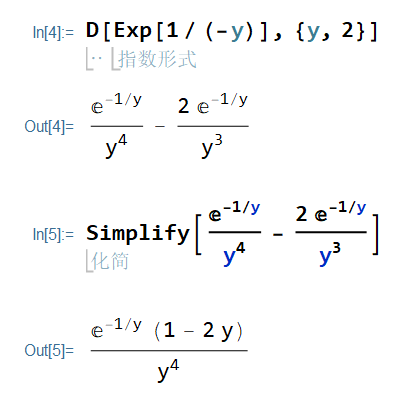# Matlab function as an objective function in CVX

I want to pass my optimization variable to a Matlab function to evaluate the objective that I want to minimize. For example

  cvx_begin
variable x(n) % My vaiable
Energy = Calculate(x,...) % Mttlab function to evaluate the objective function to be evaluated based on the variable x
minimize(Energy)
subject to
0 <= x <= 1
cvx_end


I read the two topics topic1 topic2 but is not exactly what I want to do. I appreciate if you can help. Thanks.

I did not withdraw my post. Thanks.

Create the function (make it the function you really want, provided it follows CVX 's rules):

function function_output = Calculate(x,p)
function_output = sum((x+p).^2)
end


Use it:

n = 4;
cvx_begin
variable x(n)
Energy = Calculate(x,3)
minimize(Energy)
subject to
0 <= x <= 1
cvx_end


Does this do what you want? if not, you need to be clearer as to how what you want differs from this paradigm.

1 Like

Thanks so much for your help. This is what I want. I need to modify my function to match the CVX rules. Now I have an exponential term to be calculated based on the value of x inside the function. How I should write it
p_n = b_n*exp(\frac{a*B_n*f_n}{(t_n*f_n - (A_n*x*C_n)) - 1});
Should I write the expression inside a cvx_begin cvx_end as an unconstrainted optimization?

Error using .* (line 173)
Disciplined convex programming error:
Cannot perform the operation: {positive constant} ./ {real affine}

Error in ./ (line 19)
z = times( x, y, ‘./’ );

Error in * (line 36)
z = feval( oper, x, y );

Error in / (line 15)
z = mtimes( x, y, ‘rdivide’ );

Error in Energy_Sub (line 5)
p_n = (b_n*(exp(aB_nf_n/(t_nf_n - (A_nx*C_n))) - 1));

It might not be convex if x>0. I calculated the second derivative of a simple version of yours:But if t_n*f_n - (A_n*x*C_n)) - 1 > 0, you can let y==t_n*f_n - (A_n*x*C_n)) - 1 , then express it as exp(a*B_n*f_n*inv_pos(y)) if a*B_n*f_n>0.

Thanks for your help. I have other assumptions on the values so I am sure it is convex. I used your suggestion inv_pos and it works but I have another expression

p_{n'} = b_{n'}*\exp(\frac{a*B_n*f_n}{(t_n*f_n - (A_n*x*C_n))})*(\exp(\frac{a*B_{n'}*f_{n'}}{(t_{n'}*f_{n'} - (A_{n'}*x*C_{n'}))}) - 1)

when I tried to use the same method, it produces a disciplined error. I appreciate if you can help.

Error using .* (line 173)
Disciplined convex programming error:
Cannot perform the operation: {log-convex} .* {convex}

Error in * (line 36)
z = feval( oper, x, y );

Error in Energy_Sub_CVX (line 7)
p_nn = (b_nnexp(aB_nf_ninv_pos(y_n))(exp(aB_nnf_nninv_pos(y_nn)) - 1));

can you show why this is convex?

For the first term, I have a,B_n,f_n,t_n,f_n,A_n,x,C_n >0 and the term t_n f_n - A_n x C_n > 0.
For the second term I have a,B_{n'},f_{n'},t_{n'},f_{n'},A_{n'},x,C_{n'} >0 and the term t_{n'} f_{n'} - A_{n'} x C_{n'}> 0 and the term \exp(\frac{aB_{n'}f_{n'}}{t_{n'} f_{n'}- (A_{n'}xC_{n'})} ) - 1 represents the power in my formulation and I have a constraint that it is greater than zero. I wrote the complete proof for the convexity.

I can’t understand your proof. I don’t know how to express even when it is covex. Let’s consider some very simple cases, try express exp(1/x)*(exp(1/x)-1) first, then exp(1/x)*(exp(1/(x+1))-1).

I meaned I can’t even figure out how to express something simple like exp(1/x)*(exp(1/x)-1), let alone b_{n'}*\exp(\frac{a*B_n*f_n}{(t_n*f_n - (A_n*x*C_n))})*(\exp(\frac{a*B_{n'}*f_{n'}}{(t_{n'}*f_{n'} - (A_{n'}*x*C_{n'}))}) - 1)

Thanks so much for your help. I appreciate. I will try to find a numerical search method to use instead of CVX.